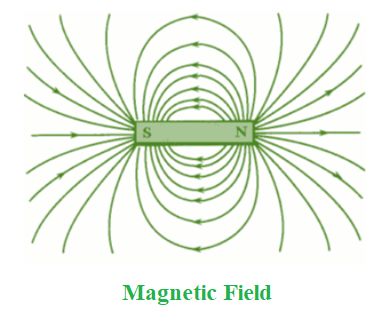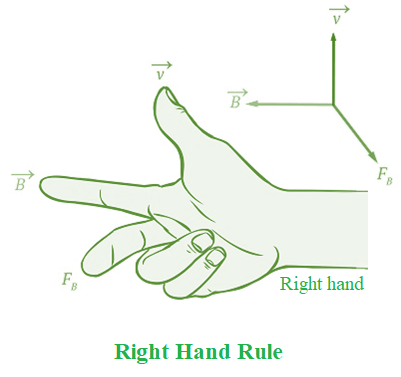Open in App
Not now

# What is Magnetism? Definition, Field, Force, Properties

• Last Updated : 05 Oct, 2021

‘Opposites attract,’ as the saying goes. You’ve probably heard this phrase a lot. But what is it that makes opposites attract? Where did this term come from? This expression is derived from the magnet and its magnetic field. A magnet’s opposing poles attract one other. In reality, shouldn’t opposing poles repel and like poles attract? Isn’t that right? ‘No,’ you say. So, let us put your theory to the test.

A magnetic field is the unseen space that exists around a magnetic object. A magnetic field describes the distribution of magnetic force around a magnetic object.

Magnetic fields are formed or produced when an electric charge/current flows near a magnet. Subatomic particles having a negative charge, such as electrons, travel about, producing a magnetic field. These fields can emerge from the atoms of magnetic objects or from electrical cables or wires.

### What is Magnetism?

A bar magnet pulls iron things to its poles, which are the ends of the magnet. The north pole is at one end, while the south pole is at the other. Magnetism is a phenomenon induced by the force exerted by magnets, which produces fields that attract or repel other metallic objects. It occurs as a result of electrically charged particles. The force acting on electrically charged particles in a magnetic field is determined by the amount of the charge, the particle’s velocity, and the strength of the field. According to magnetism:

• Like poles repel each other.
• Opposite poles attract each other.

When two bar magnets are brought close together, the like poles repel each other while the unlike poles attract.

### Magnetic field

When a positively charged particle moves in a homogeneous magnetic field, the particle’s velocity is perpendicular to the field. The magnetic force always operates in a direction perpendicular to the charge’s motion.

The magnetic field is the space or region around a magnet in which magnetic force is applied on another magnet.

The magnetic field can be represented in a variety of ways. It may be represented mathematically as a vector field that can be displayed as various sets on a grid. Another method is to utilise field lines. Lines link the vectors in the set. Magnetic field lines never cross one other and never come to a halt here.A magnetic compass is used to locate the magnetic field of a bar magnet. A magnetic compass does not deflect when maintained away from a magnet. When you bring a magnetic compass closer to a magnet, it deflects. If the magnetic field lines in a specific location are very near to one other, the magnetic field is very strong; if the magnetic field lines are very far apart, the magnetic field is very weak. It is denoted by the unit Tesla.

Magnetic Field Unit and Measurement

The magnetic field is measured by determining its intensity and direction. The measurement is required because each magnetic field differs from the others. Magnetic field intensity varies from tiny and weak to extremely powerful and huge. When we look at the Earth’s magnetic field, we can see that it is faint yet significant.

Nonetheless, the word magnetic field refers to two distinct but related fields, represented by the symbols H and B. H is the magnetic field strength and is measured in amperes per metre, the SI unit. Meanwhile, B denotes magnetic flux density and is expressed in tesla.

### Magnetic Field Lines

Field lines are another method to display the information contained inside a magnetic vector field. Magnetic field lines are fictitious lines.

Magnetic field lines are a type of visual representation of magnetic fields. They describe the magnetic force direction on a north monopole at any particular point.

The magnitude of the field is indicated by the density of the lines. For example, at the poles of a magnet, the magnetic field is stronger and denser. It becomes weaker as we go away from the poles, and the lines become less thick.

Magnetic Field Line Properties

• Magnetic field lines never cross one other.
• Magnetic field lines are always closed loops.
• The density of the field lines reflects the field’s intensity.
• Magnetic field lines always arise from or begin at the north pole and end at the south pole.

### Magnetic force

The magnetic force is the attraction or repulsion force that exists between electrically charged particles as a result of their motion.

The magnetic force between two moving charges is defined as the force imposed on one’s charge by the magnetic field generated by the other. This force is responsible for magnets attracting or repelling one another.

A compass, a motor, the magnets that keep things on the refrigerator, railway lines, and new roller coasters are all examples of magnetic force. A magnetic field is created by all moving charges, and the charges that travel across its areas experience a force. Depending on whether the force is attractive or repulsive, it might be positive or negative. The magnetic force of an item is determined by its charge, velocity, and magnetic field.

Force on a moving charge

A charge will feel a force if it travels at an angle across a magnetic field. The equation is:

F = q v B sin θ

Here,

• q is the charge,
• B is the magnetic field,
• v is the velocity, and
• θ is the angle between the magnetic field and velocity directions.

A force acts on the motion of charge q travelling with velocity v in a magnetic field, and this force is:

1. perpendicular to sinθ where θ denotes the angle formed by v and B
2. proportional to the charge q
3. perpendicular to both B and v
4. proportional to the velocity v

### Right-Hand Rule

The Right-hand rule can be used to determine the force direction (F).

It refers to devices that create currents by moving in a magnetic field.

• Point your pointer finger towards the direction of charge v’s motion.
• Between v and B, rotate your middle finger away from your index finger.
• Hold your thumb parallel to the plane produced by your index and middle fingers.
• If the charge q is positive, your thumb will point in the direction of the force (F).### Sample Questions

Question 1: What is the magnetic force of 40 C charged particles travelling at 5 m/s in a magnetic field of 2 T? Its field’s direction is the same as the route of the second particle.

Given:

Charge, q = 40 C

Velocity, v = 5 m ⁄ s

Magnetic Field, B = 2 T

Because the second particle’s route difference is the same as the direction of its field, θ =0°

The force, F= q v B sin θ = 40 × 3 × 1 × sin 0° = 0.

Hence, the magnetic force of charged particles is 0 N.

Question 2: What exactly is magnetic force?

The magnetic force between two moving charges can be defined as the impact of one charge’s magnetic field on the other.

Question 3: What is the significance of magnetic flux lines?

Magnetic flux lines are significant for the following reasons:

• They indicate the magnetic field’s direction.
• The intensity of a magnetic field is proportional to the number of magnetic field lines present in a given region.
• Magnetic field lines are lines in a magnetic field whose tangent at each location indicates the field direction at that place and whose density gives the field magnitude.
• The strength of the magnetic field is proportional to the number of magnetic field lines. Because the lines are higher near the poles, the magnetic field is greater there.

Question 4: Are magnetic fields present in space?